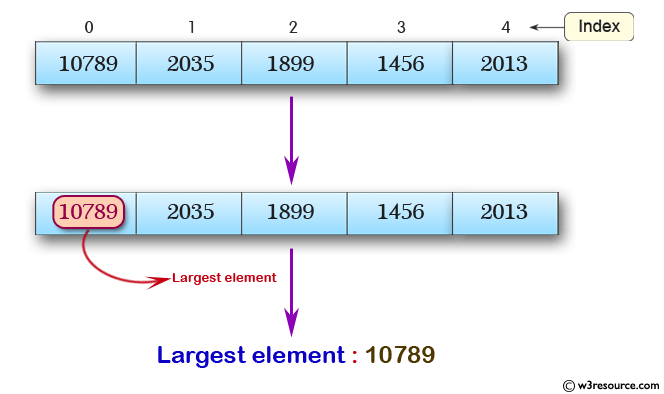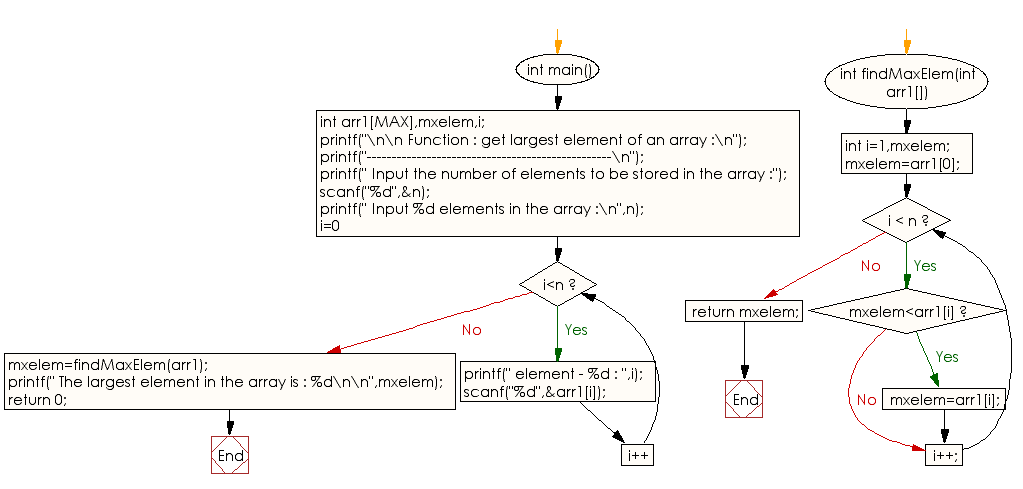﻿ C Program: Get largest element of an array - w3resource

# C Exercises: Get largest element of an array

## C Function : Exercise-8 with Solution

Write a program in C to get largest element of an array using the function.

Pictorial Presentation:Sample Solution:

C Code:

``````#include<stdio.h>
#define MAX 100

int findMaxElem(int []);
int n;

int main()
{
int arr1[MAX],mxelem,i;
printf("\n\n Function : get largest element of an array :\n");
printf("-------------------------------------------------\n");

printf(" Input the number of elements to be stored in the array :");
scanf("%d",&n);

printf(" Input %d elements in the array :\n",n);
for(i=0;i<n;i++)
{
printf(" element - %d : ",i);
scanf("%d",&arr1[i]);
}
mxelem=findMaxElem(arr1);

printf(" The largest element in the array is : %d\n\n",mxelem);
return 0;
}
int findMaxElem(int arr1[])
{
int i=1,mxelem;
mxelem=arr1;
while(i < n)
{
if(mxelem<arr1[i])
mxelem=arr1[i];
i++;
}
return mxelem;
}
```
```

Sample Output:

```Function : get largest element of an array :
-------------------------------------------------
Input the number of elements to be stored in the array :5
Input 5 elements in the array :
element - 0 : 1
element - 1 : 2
element - 2 : 3
element - 3 : 4
element - 4 : 5
The largest element in the array is : 5
```

Explanation:

```int findMaxElem(int arr1[]) {
int i = 1, mxelem;
mxelem = arr1;
while (i < n) {
if (mxelem < arr1[i])
mxelem = arr1[i];
i++;
}
return mxelem;
}
```

The function 'findMaxElem' and takes a single argument of type int array, named arr1. It finds the maximum element in the array by iterating through all elements of the array and keeping track of the maximum element seen so far.

The function initializes two local integer variables, i and mxelem, to 1 and the first element of the array arr1 respectively. It then enters a while loop that continues as long as i is less than the length of the array n.

In each iteration of the loop, the function compares the current element of the array arr1[i] with the current maximum element mxelem. If arr1[i] is greater than mxelem, mxelem is updated to the value of arr1[i]. The loop continues until all elements of the array have been processed. Finally, the function returns the maximum element 'mxelem'.

Time complexity and space complexity:

The time complexity of this function is O(n), where n is the length of the input array arr1, because the while loop iterates n-1 times to find the maximum element of the array. The space complexity of this function is O(1), as it only uses a fixed amount of memory to store the three integer variables i, mxelem, and the input array arr1.

Flowchart:C Programming Code Editor:

What is the difficulty level of this exercise?

Test your Programming skills with w3resource's quiz.

﻿